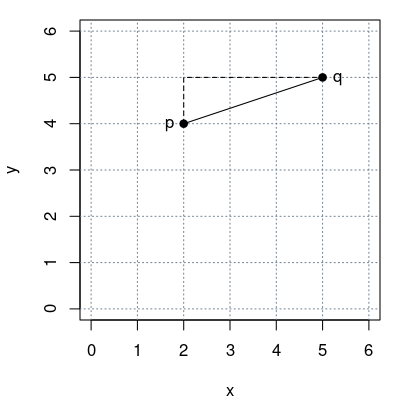## Exam 1

1. #### Question

What is the distance between the two points $p = (2, 4)$ and $q = (5, 5)$ in a Cartesian coordinate system?

1. $1.261$
2. $2.000$
3. $2.695$
4. $0.408$
5. $3.162$

#### Solution

The distance $d$ of $p$ and $q$ is given by $d^2 = (p_1 - q_1)^2 + (p_2 - q_2)^2$ (Pythagorean formula).

Hence $d = \sqrt{(p_1 - q_1)^2 + (p_2 - q_2)^2} = \sqrt{(2 - 5)^2 + (4 - 5)^2} = 3.162$.1. False
2. False
3. False
4. False
5. True